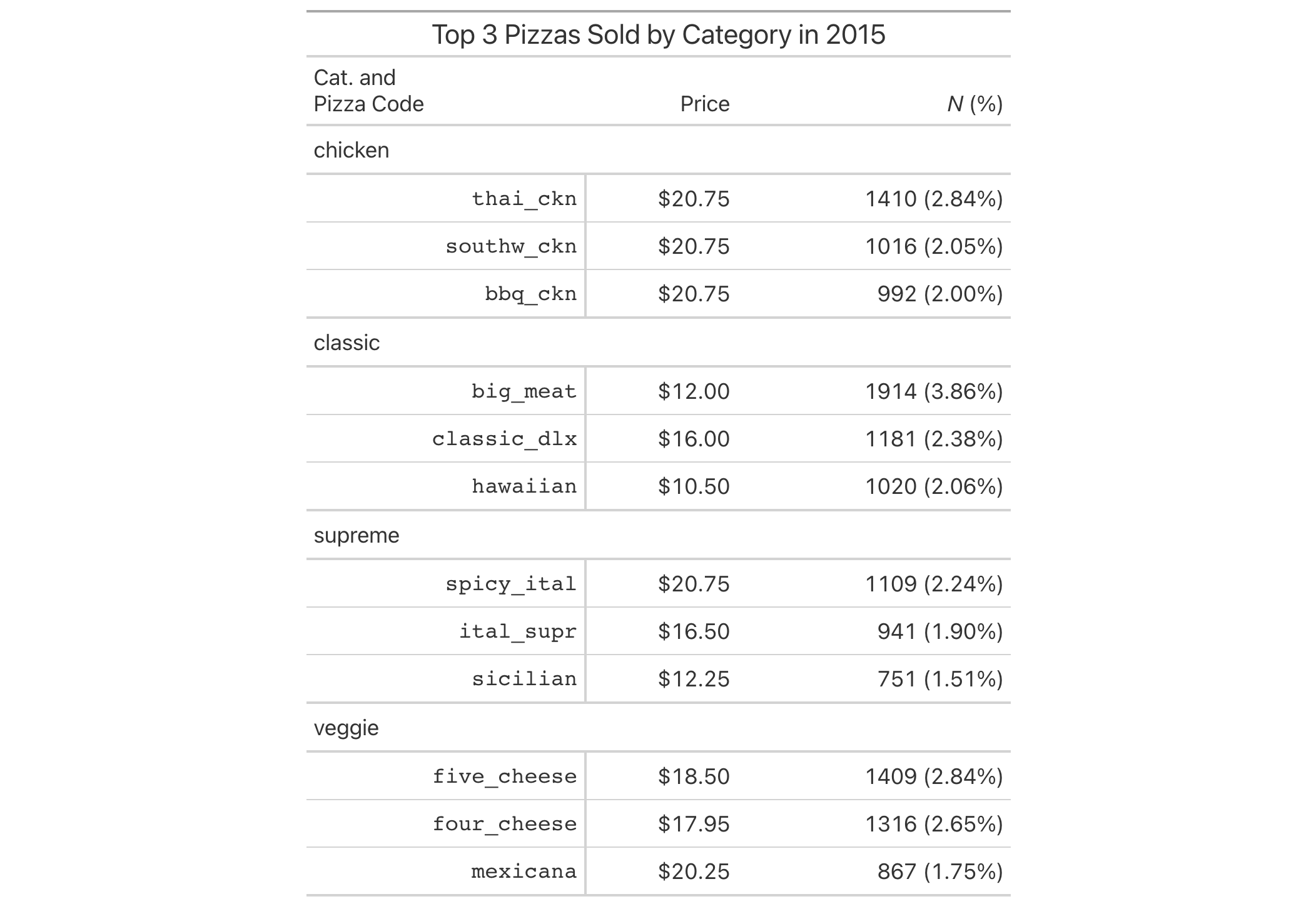The cols_merge_n_pct() function is a specialized variant of the cols_merge() function. It operates by taking two columns that constitute both a count (col_n) and a fraction of the total population (col_pct) and merges them into a single column. What results is a column containing both counts and their associated percentages (e.g., 12 (23.2%)). The column specified in col_pct is dropped from the output table.

## Usage

cols_merge_n_pct(data, col_n, col_pct, autohide = TRUE)

## Arguments

data

A table object that is created using the gt() function.

col_n

A column that contains values for the count component.

col_pct

A column that contains values for the percentage component. This column should be formatted such that percentages are displayed (e.g., with fmt_percent()).

autohide

An option to automatically hide the column specified as col_pct. Any columns with their state changed to hidden will behave the same as before, they just won't be displayed in the finalized table.

## Value

An object of class gt_tbl.

## Details

This function could be somewhat replicated using cols_merge(), however, cols_merge_n_pct() employs the following specialized semantics for NA and zero-value handling:

1. NAs in col_n result in missing values for the merged column (e.g., NA + 10.2% = NA)

2. NAs in col_pct (but not col_n) result in base values only for the merged column (e.g., 13 + NA = 13)

3. NAs both col_n and col_pct result in missing values for the merged column (e.g., NA + NA = NA)

4. If a zero (0) value is in col_n then the formatted output will be "0" (i.e., no percentage will be shown)

Any resulting NA values in the col_n column following the merge operation can be easily formatted using the sub_missing() function. Separate calls of sub_missing() can be used for the col_n and col_pct columns for finer control of the replacement values. It is the responsibility of the user to ensure that values are correct in both the col_n and col_pct columns (this function neither generates nor recalculates values in either). Formatting of each column can be done independently in separate fmt_number() and fmt_percent() calls.

This function is part of a set of four column-merging functions. The other two are the general cols_merge() function and the specialized cols_merge_uncert() and cols_merge_range() functions. These functions operate similarly, where the non-target columns can be optionally hidden from the output table through the hide_columns or autohide options.

## Examples

Use pizzaplace to create a gt table that displays the counts and percentages of the top 3 pizzas sold by pizza category in 2015. The cols_merge_n_pct() function is used to merge the n and frac columns (and the frac column is formatted using fmt_percent()).

pizzaplace %>%
dplyr::group_by(name, type, price) %>%
dplyr::summarize(
n = dplyr::n(),
frac = n/nrow(.),
.groups = "drop"
) %>%
dplyr::arrange(type, dplyr::desc(n)) %>%
dplyr::group_by(type) %>%
gt(
rowname_col = "name",
groupname_col = "type"
) %>%
fmt_currency(price) %>%
fmt_percent(frac) %>%
cols_merge_n_pct(
col_n = n,
col_pct = frac
) %>%
cols_label(
n = md("*N* (%)"),
price = "Price"
) %>%
tab_style(
style = cell_text(font = "monospace"),
locations = cells_stub()
) %>%
tab_options(table.width = px(512))Other column modification functions: cols_align_decimal(), cols_align(), cols_hide(), cols_label(), cols_merge_range(), cols_merge_uncert(), cols_merge(), cols_move_to_end(), cols_move_to_start(), cols_move(), cols_unhide(), cols_width()